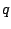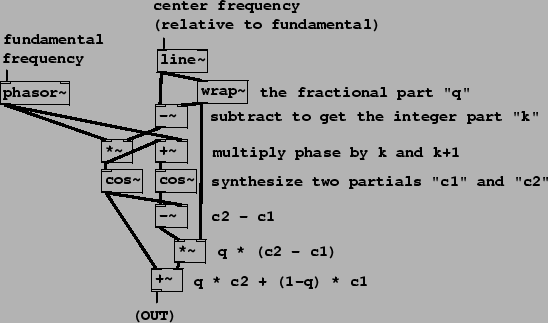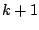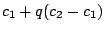Next: The PAF generator Up: Examples Previous: Simple formant generator   Contents   Index

## Two-cosine carrier signal

Example F08.two.cosines.pd (Figure 6.17) shows how to make a carrier signal that cross-fades between harmonics to make continuously variable center frequencies. The center frequency quotient appears as the output of a line~ object. This is separated into its fractional part (using the wrap~ object) and its integer part (by subtracting the fractional part from the original). These are labeled asandto agree with the treatment in Section 6.3.The phase--a sawtooth wave at the fundamental frequency--is multiplied by bothand(the latter by adding the original sawtooth into the former), and the cosines of both are taken; they are therefore atandtimes the fundamental frequency and have no discontinuities at phase wrapping points. The next several objects in the patch compute the weighted sum, where,are the two sinusoids and, by evaluating an equivalent expression,. This gives us the desired movable-frequency carrier signal.

Example F09.declickit.pd (not shown here) shows how, by adding a samphold~ object after the line~ object controlling center frequency, you can avoid discontinuities in the output signal even if the desired center frequency changes discontinuously. In the example the center frequency quotient alternates between 4 and 13.5. At ramp times below about 20 msec there are audible artifacts when using the line~ object alone which disappear when the samphold~ object is added. (A disadvantage of sample-and-holding the frequency quotient is that, for very low fundamental frequencies, the changes can be heard as discrete steps. So in situations where the fundamental frequency is low and the center frequency need not change very quickly, it may be better to omit the sample-and-hold step.)

The next two examples demonstrate using the crossfading-oscillators carrier as part of the classic two-operator phase modulation technique. The same modulating oscillator is added separately to the phases of the two cosines. The resulting spectra can be made to travel up and down in frequency, but because of the complicated phase relationships between neighboring peaks in the phase modulation spectrum, no matter how you align two such spectra you can never avoid getting phase cancellations where they overlap.Next: The PAF generator Up: Examples Previous: Simple formant generator   Contents   Index
Miller Puckette 2006-12-30